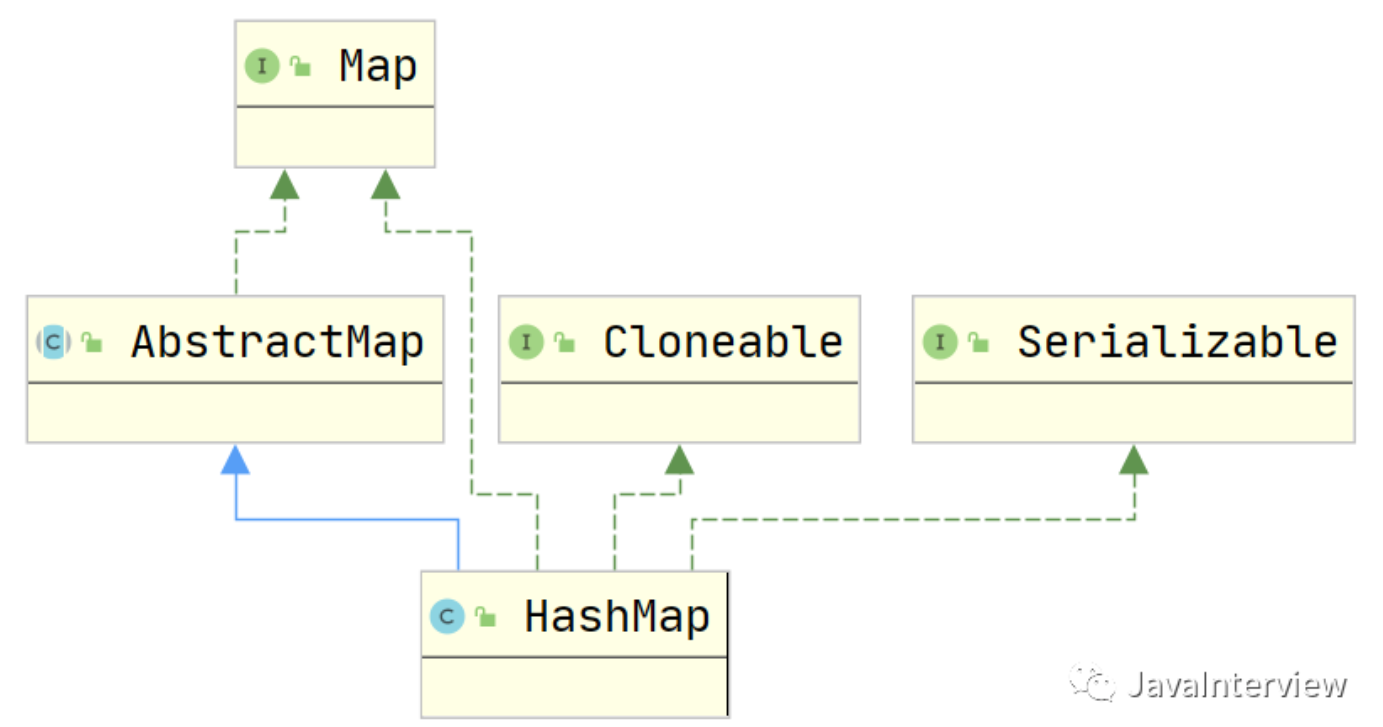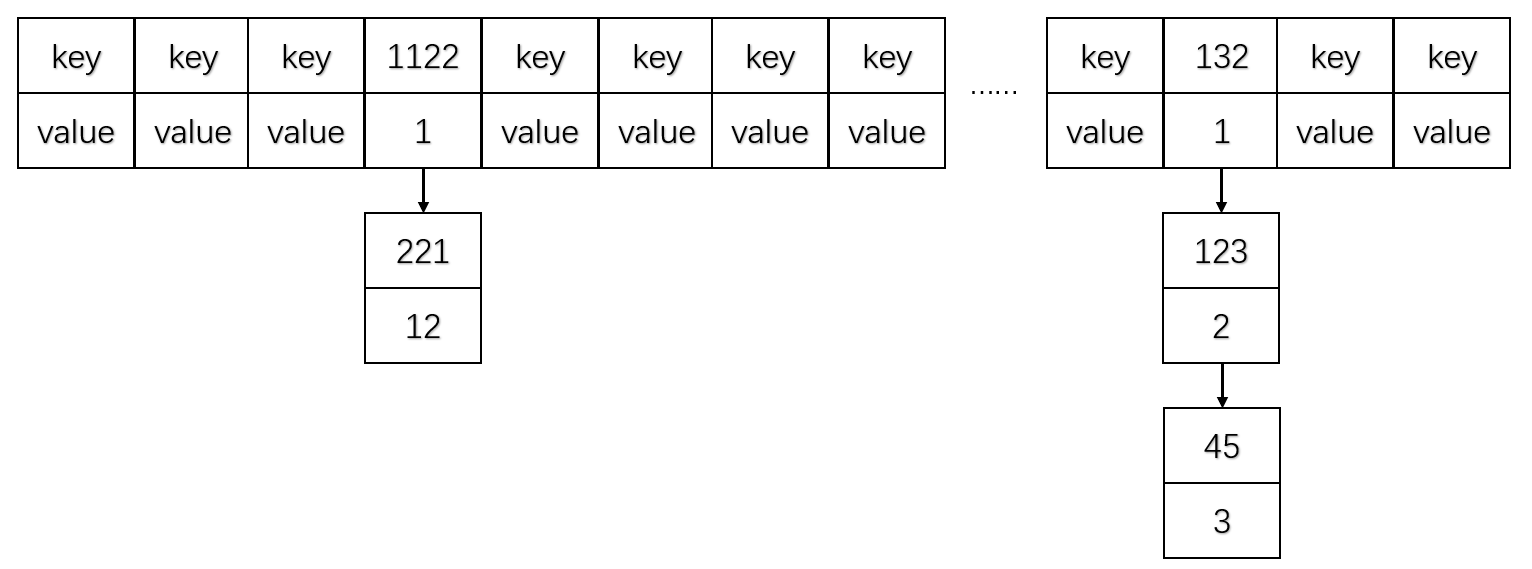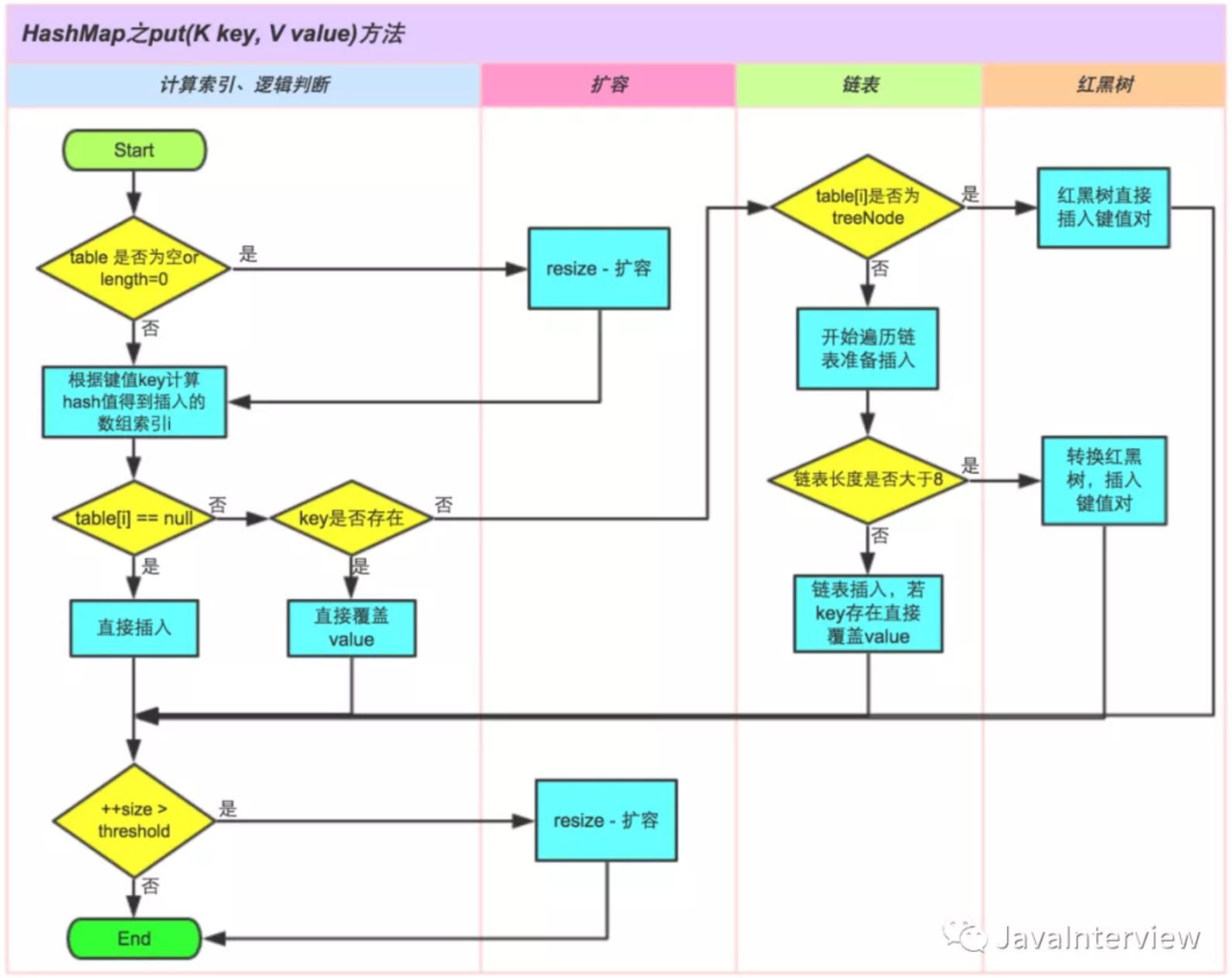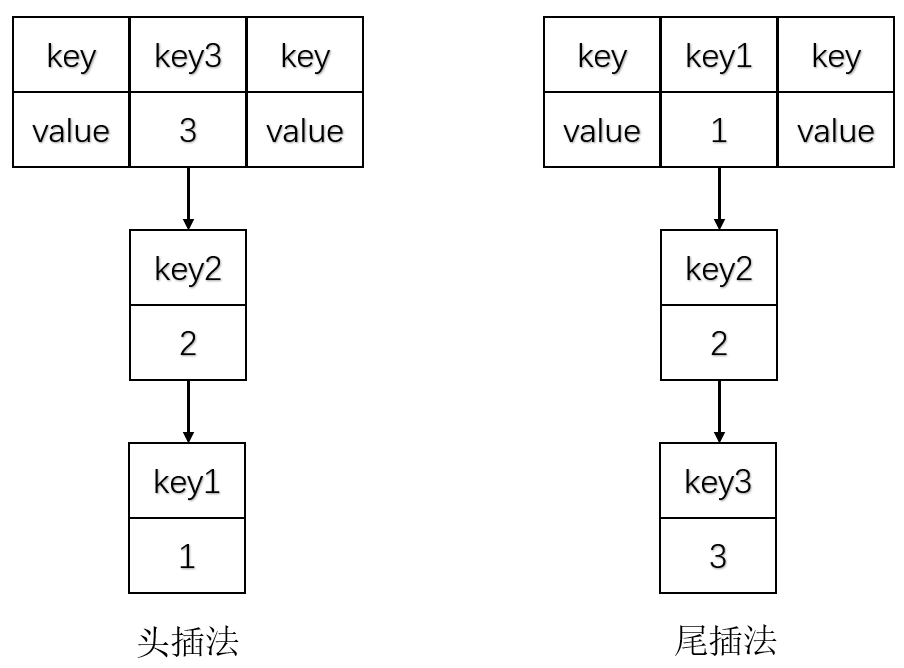# HashMap摘要

HashMap应该算是Java程序员使用频率最高的容器类了，它主要用于处理键值映射数据。随着JDK的升级，JDK1.8后对HashMap底层采取了不少优化，例如引入红黑树，改用Node类存放节点等。但HashMap是线程不安全的类，遇到多线程问题时需要改用其他相关线程安全类。

# HashMap继承体系

HashMap<K,V>是一个key-value结构，继承自抽象类AbstractMap<K,V>，实现了Map<K,V>以及Cloneable和Serializable接口；Cloneable是一个标记接口，里面没有任何东西，表明类中需要重写clone()方法时必须实现此接口；Serializable也是一个标记接口，表明需要序列化此类对象时必须实现该接口。# HashMap容量

HashMap初始化容量DEFAULT_INITIAL_CAPACITY = 16，默认不写容量时就是16，下列是源码写法。首先，为何初始容量选择16，这个没有太多的解释，经验表明，16应该是大多数开发者最好的选择；其次，为何写成1 << 4，而不是直接写16，这个里面原理就深入了。

``````static final int DEFAULT_INITIAL_CAPACITY = 1 << 4; // aka 16
``````

``````index = (Length - 1) & hash
``````

resize扩容有两个因素，一是HashMap当前长度capacity，二是负载因子，默认为0.75；当存入的数据大于当前长度乘以负载因子时就要进行扩容。

``````static final float DEFAULT_LOAD_FACTOR = 0.75f;
``````

``````static final int MAXIMUM_CAPACITY = 1 << 30;

static final int TREEIFY_THRESHOLD = 8;

static final int UNTREEIFY_THRESHOLD = 6;

static final int MIN_TREEIFY_CAPACITY = 64;
``````

# HashMap数据结构

JDK1.7和JDK1.8发生了一些变化。HashMap主要由数组和链表组成，具体结构如下图所示。在Java7中每个位置叫Entry，Java8中叫Node，每个位置下面还连着链表，当然，Java8后链表超过一定容量（容量为8）就自动转化为红黑树。

``````static class Node<K,V> implements Map.Entry<K,V> {
final int hash;
final K key;
V value;
HashMap.Node<K, V> next;
}
``````

HashMap中有几个关键字段，用于初始化HashMap默认构造函数。

``````transient int size;
transient int modCount;
int threshold;            // 所能容纳的key-value对极限
final float loadFactor;  // 负载因子
``````

``````static final int hash(Object key) {
int h;
return (key == null) ? 0 : (h = key.hashCode()) ^ (h >>> 16);
}
``````

HashMap在进行put操作时，先计算出key对应的hash值，根据hash值做位与运算得到对应的index。我们知道，HashMap容量有限，不同的key的hash值会存在碰撞（hash碰撞具体原因查看数据结构中对于Hash的讲解），解决hash碰撞的一个方法就是使用拉链法，具体操作就是将相同hash值的节点形成链表。拉链法形成方式如下图。（1）判断键值对数组table[i]是否为空或null，否则执行resize()进行扩容；

（2）根据键值key计算hash值得到插入的数组索引i，如果table[i]==null，直接新建节点添加，转向（6），如果table[i]不为空，转向（3）；

（3）判断table[i]的首个元素是否和key一样，如果相同直接覆盖value，否则转向（4），这里的相同指的是hashCode以及equals；

（4）判断table[i]是否为treeNode，即table[i]是否是红黑树，如果是红黑树，则直接在树中插入键值对，否则转向（5）；

（5）遍历table[i]，判断链表长度是否大于8，大于8的话把链表转换为红黑树，在红黑树中执行插入操作，否则进行链表的插入操作；遍历过程中若发现key已经存在直接覆盖value即可；

（6）插入成功后，判断实际存在的键值对数量size是否超多了最大容量threshold，如果超过，进行扩容。

JDK1.8HashMap中put源码如下。

``````public V put(K key, V value) {
return putVal(hash(key), key, value, false, true);
}

final V putVal(int hash, K key, V value, boolean onlyIfAbsent,
boolean evict) {
Node<K,V>[] tab; Node<K,V> p; int n, i;
// （1）tab为空则创建
if ((tab = table) == null || (n = tab.length) == 0)
n = (tab = resize()).length;
// （2）计算index，并对null处理
if ((p = tab[i = (n - 1) & hash]) == null)
tab[i] = newNode(hash, key, value, null);
else {
Node<K,V> e; K k;
// 节点key存在，直接覆盖value
if (p.hash == hash &&
((k = p.key) == key || (key != null && key.equals(k))))
e = p;
// 判断该链为红黑树
else if (p instanceof TreeNode)
e = ((TreeNode<K,V>)p).putTreeVal(this, tab, hash, key, value);
else {
for (int binCount = 0; ; ++binCount) {
if ((e = p.next) == null) {
p.next = newNode(hash, key, value, null);
// 链表大于8转换为红黑树
if (binCount >= TREEIFY_THRESHOLD - 1) // -1 for 1st
treeifyBin(tab, hash);
break;
}
// key已经存在覆盖value
if (e.hash == hash &&
((k = e.key) == key || (key != null && key.equals(k))))
break;
p = e;
}
}
if (e != null) { // existing mapping for key
V oldValue = e.value;
if (!onlyIfAbsent || oldValue == null)
e.value = value;
afterNodeAccess(e);
return oldValue;
}
}
++modCount;
// 超过最大容量就扩容
if (++size > threshold)
resize();
afterNodeInsertion(evict);
return null;
}
``````HashMap在形成链表进行插入时，在Java8之前采用头插法，而Java8之后采用尾插法，头插法和尾插法比较如下图，插入顺序为key1-key2-key3。# HashMap线程安全性问题

HashMap是线程不安全的，它的每个方法都没有涉及到加锁机制，在多线程环境下，很容易造成线程不安全。

``````Map<String, String> map = new Hashtable<>();

Map<String, String> map = new HashMap<>();
Map<String, String> map1 = Collections.synchronizedMap(map);

Map<String, String> map = new ConcurrentHashMap<>();
``````

end
• 作者：JJ（联系作者）
• 发表时间：2021-02-15 13:04
• 版权声明：自由转载-非商用-非衍生-保持署名（创意共享3.0许可证）
• 转载声明：如果是转载博主转载的文章，请附上原文链接
• 公众号转载：请在文末添加作者公众号二维码（公众号二维码见右边，欢迎关注）
•